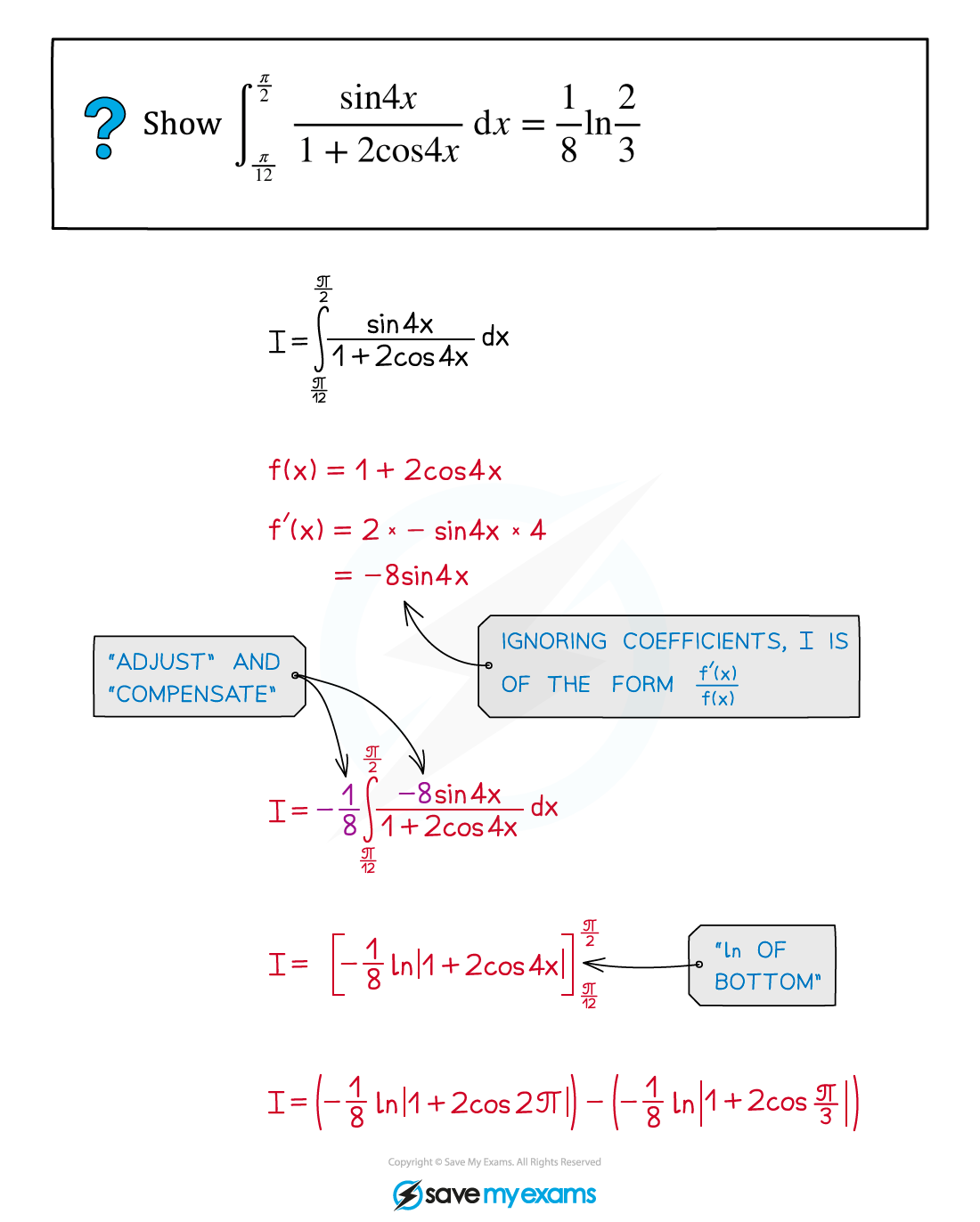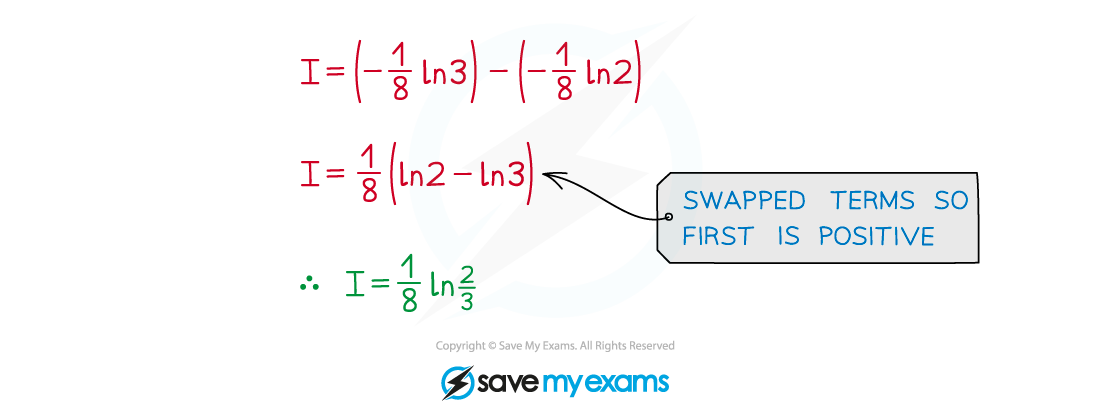# AQA A Level Maths: Pure复习笔记8.2.4 f'(x)/f(x)

### f'(x)/f(x)

#### Integrating fractions

• The technique for integrating fractions depends on the type of fraction
• For polynomial denominators see Integration using Partial Fractions
•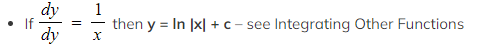The type of fraction dealt with here is a specific case of Reverse Chain Rule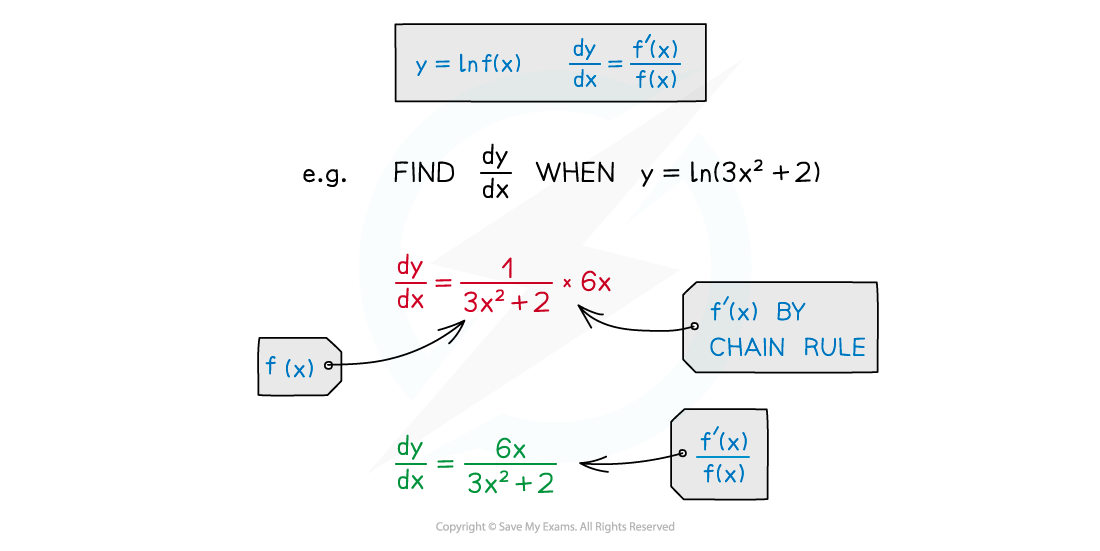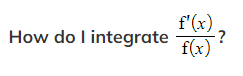• “The top is ‘almost’ the derivative of the bottom”
• 'almost' here meaning 'a multiple of' (see below)
• The integral will involve ln |f(x)| - ie ln of the bottom
• Due to reverse chain rule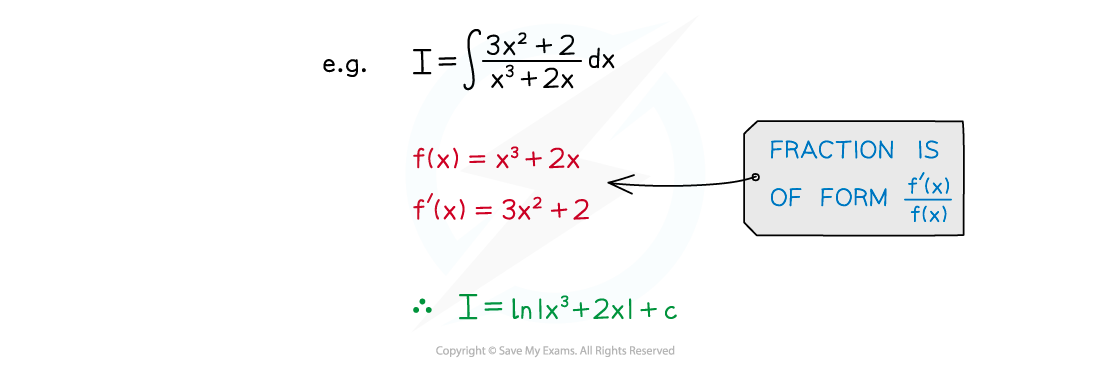#### Why ‘almost’?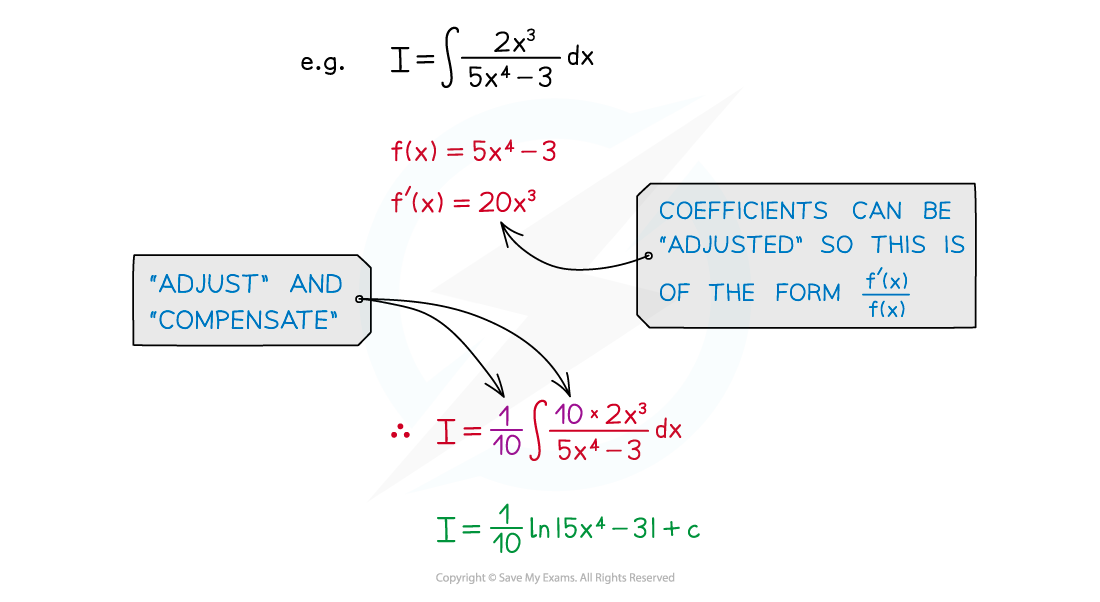• There may be coefficients to ‘adjust’ and ‘compensate’ for

#### Exam Tip

• If you're unsure if the fraction is of the form f’(x)/f(x), differentiate the denominator.
• Compare this to the numerator but you can ignore any coefficients.
• If the coefficients do not match then ‘adjust’ and ‘compensate’ for them.

#### Worked Example